Definitions of Square Dance Calls and Concepts

\$BJ?9T\$J(B Line \$B\$+\$i!%(B

1. Explode;
2. End Beau Circulate 1 & 1/2 as End Belle Circulate, Veer inward, and U-Turn Back as Centers Touch 1/2 and the Very Centers Cast Off 3/4 while the other Center Step Ahead to form a R-H Star;
3. Turn the Star 1/4;
4. The End of the Center Wave walks forward as everyone else follows to Unwrap The Star, and everyone Face In to end in Facing Lines.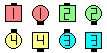Explode The Top\$B\$NA0(B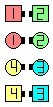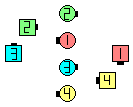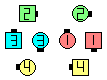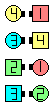Explode\$B\$N8e(B Ends move to their Star position, as Centers Touch 1/2\$B\$N8e(B Very Centers Cast Off 3/4 remaining Centers Step Ahead(\$B\$9\$J\$o\$A(B, turn the Star \$B\$NA0(B) \$B\$N8e(B Star 1/4; Very Outsides lead Unwrap The Star,\$B\$=\$7\$F(B Face In \$B\$N8e(B (\$B=*\$o\$j(B)

\$BCm
• The Center who Stepped Ahead should raise their Left hand to indicate that they are leading the Unwrap The Star;
• The leader of the Unwrap is the End of the Center Wave after th star has turned 1/4.
• \$B%@%s%9\$N%R%s%H(B: Unwrap \$B\$N\$H\$-\$N(B very ends (\$B>e\$N?^\$G(B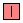\$B\$H(B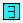\$B\$N?M(B) \$B\$O(B Unwrap \$B\$H(B 1/4 In \$B\$NBe\$o\$j\$K(B, Star 1/2, \$BA0?J\$7(B, \$BNY\$N?M\$H>e\$2\$kOS\$N2<\$G(B 1/4 \$B:8\$r8~\$/\$h\$&\$KF0\$/(B (Circle To A Line [B] \$B\$G\$N%*%j%8%J%k\$N30B&\$N(B Belle \$B\$,9T\$&\$h\$&\$K(B) \$B\$3\$H\$,\$G\$-\$^\$9!%(B
• \$B1~MQ(B: Explode The Top turn the Star n less (or more) position(s)\$B!%(BStar \$B\$G2s\$C\$?\$"\$H(B, Center Wave \$B\$N(B ends \$B\$K\$J\$C\$??M\$,(B, \$B>o\$K(B Unwrap \$B\$N%j!<%@\$K\$J\$j\$^\$9!%(B